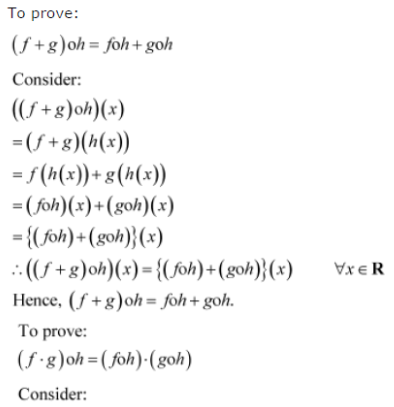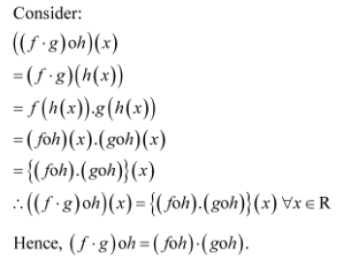# Let f, g and h be functions from R to R. Show that (f + g)oh = foh + goh (f . g)oh = (foh) . (goh)

Let f, g and h be functions from R to R. Show that
(f + g)oh = foh + goh
(f . g)oh = (foh) . (goh)